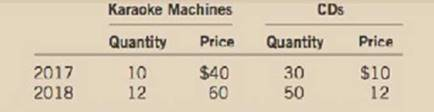Chapter 6, Problem 5PA

Chapter
Section
Textbook Problem

A small nation of ten people idolizes the TV show The Voice. All they produce and consume are karaoke machines and CDs, in the following amounts:a. Using a method similar to the CPI, compute the percentage change in the overall price level. Use 2017 as the base year and fix the basket at 1 karaoke machine and 3 CDs.b. Using a method similar to the GUP deflator, compute the percentage change in the overall price level. Also use 2017 as the base year.c. Is the inflation rate in 2018 the same using the two methods? Explain why or why not.

Subpart (a):

To determine

Measuring percentage change in price using CPI and GDP deflator.

Explanation

Cost of market for the basket can be calculated by using the following formula.

Cost of Market basket=((Price1×Quantity1)+(Price2×Quantity2)+...+(Pricen×Quantityn)) (1)

Substitute the respective values in Equation (2) to calculate the cost of market basket in the year 2017:

Cost of the market basket2017=1×40+3×10=70

Cost of market basket for the year 2017 is $70. Substitute the respective values in Equation (2) to calculate the cost of market basket in the year 2018: Cost of the market basket2018=1×60+3×12=96 Cost of market basket for the year 2018 is$96

Subpart (b):

To determine

Measuring percentage change in price using CPI and GDP deflator.

Subpart (c):

To determine

Measuring percentage change in price using CPI and GDP deflator.

Still sussing out bartleby?

Check out a sample textbook solution.

See a sample solution

The Solution to Your Study Problems

Bartleby provides explanations to thousands of textbook problems written by our experts, many with advanced degrees!

Get Started

Explain why economists usually oppose controls on prices.

Principles of Macroeconomics (MindTap Course List)

How does capitalism differ from socialism and communism?

Foundations of Business (MindTap Course List)

INCOME STATEMENT Hermann Industries is forecasting the following income statement: Sales 8,000,000 Operating co...

Fundamentals of Financial Management, Concise Edition (with Thomson ONE - Business School Edition, 1 term (6 months) Printed Access Card) (MindTap Course List)